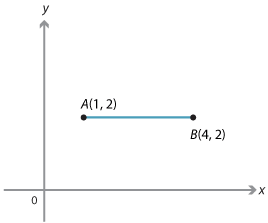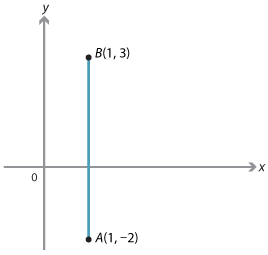## Content description

Find the distance between two points located on a Cartesian plane using a range of strategies, including graphing software (ACMNA214)

Source: Australian Curriculum, Assessment and Reporting Authority (ACARA)

### Distance between two points

Distances are always positive, or zero if the points coincide. The distance from A to B is the same as the distance from B to A.
We first find the distance between two points that are either aligned vertically or horizontally.

#### Example 1

Find the distance between the following pairs of points:

1. A(1, 2) and B(4, 2)
2. A(1, −2) and B(1, 3)

#### Solution

1. The distance AB = 4 − 1 = 3.Note: The distance AB is obtained from the difference of the x-coordinates of the two points.
2. The distance AB = 3 − (−2) = 5.Note: The distance AB is obtained from the difference of the y-coordinates of the two points.